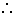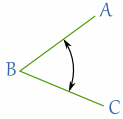# Symbols in Geometry

## Common Symbols Used in Geometry

Symbols save time and space when writing. Here are the most common geometrical symbols:

Symbol Meaning Example In Words
Triangle △ABC has 3 equal sides Triangle ABC has three equal sides
Angle ∠ABC is 45° The angle formed by ABC is 45 degrees.
Perpendicular AB⊥CD The line AB is perpendicular to line CD
Parallel EF∥GH The line EF is parallel to line GH
° Degrees 360° 360 degrees (a full rotation!)
∟  Right Angle (90°) ∟ is 90° A right angle is 90 degreesLine Segment "AB" AB The line segment between A and BLine "AB"The infinite line that includes A and BRay "AB"The line that starts at A, goes through B and continues on
Congruent (same shape and size) △ABC ≅ △DEF
Triangle ABC is congruent to triangle DEF
Similar (same shape, different size) △DEF∼△MNO Triangle DEF is similar to triangle MNO
Therefore a=bb=a a equals b, therefore b equals a

### Example: In △ABC, ∠BAC is ∟

Is really saying: "In triangle ABC, the angle BAC is a right angle"### Naming Angles

For angles the central letter is where the angle is.

### Example: ∠ABC is 45°

The point "B" is where the angle is.

3262, 3263, 3264, 3265, 3266, 3267, 3268, 3269, 5154, 5155